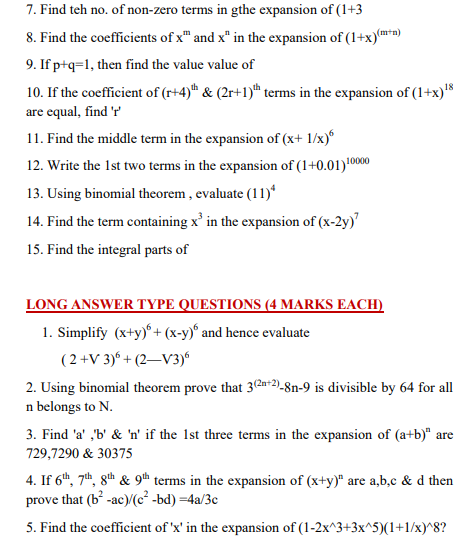# Binomial Theorem Class 11 Concepts notes

• ### CBSE Class 11 Mathematics Binomial Theorem Notes

Class XI: Chapter 8: Binomial Theorem Chapter Notes Key Concepts 1. A binomial expression is an algebraic expression having two terms, for example (a+b),(a-b) etc. Please click the link below to download pdf file for CBSE Class 11 Mathematics - Binomial Theorem Concepts.

• ### CBSE Class 11 Mathematics Binomial Theorem Formulas And Questions

Please refer to attached file for CBSE Class 11 Maths Binomial Theorem Formulaes and Questions

# CBSE Class 11 Mathematics Binomial Theorem Notes

Class XI:

Chapter 8: Binomial Theorem

Click for more Mathematics Study Material
 CBSE Class 11 Mathematics Set Theory Notes
 CBSE Class 11 Mathematics Relations And Functions Notes
 CBSE Class 11 Mathematics Complex Numbers Quadratic Equations Notes
 CBSE Class 11 Mathematics Linear Inequalities Notes
 CBSE Class 11 Mathematics Permutation And Combination Formulas And Questions CBSE Class 11 Mathematics Permutation And Combination Notes
 CBSE Class 11 Mathematics Sequence And Series Formulas And Questions CBSE Class 11 Mathematics Sequence And Series Notes
 CBSE Class 11 Mathematics Straight Lines Formulas And Questions
 CBSE Class 11 Mathematics Coordinate Geometry Formulas And Questions CBSE Class 11 Mathematics Three Dimensional Geometry Notes
 CBSE Class 11 Mathematics Limits And Derivatives Notes
 CBSE Class 11 Mathematics Mathematical Reasoning Notes
 CBSE Class 11 Mathematics Statistics Notes
 CBSE Class 11 Mathematics Probability Notes

# CBSE Class 11 Mathematics Binomial Theorem Formulas And QuestionsClick for more Mathematics Study Material
 CBSE Class 11 Mathematics Set Theory Notes
 CBSE Class 11 Mathematics Relations And Functions Notes
 CBSE Class 11 Mathematics Complex Numbers Quadratic Equations Notes
 CBSE Class 11 Mathematics Linear Inequalities Notes
 CBSE Class 11 Mathematics Permutation And Combination Formulas And Questions CBSE Class 11 Mathematics Permutation And Combination Notes
 CBSE Class 11 Mathematics Sequence And Series Formulas And Questions CBSE Class 11 Mathematics Sequence And Series Notes
 CBSE Class 11 Mathematics Straight Lines Formulas And Questions
 CBSE Class 11 Mathematics Coordinate Geometry Formulas And Questions CBSE Class 11 Mathematics Three Dimensional Geometry Notes
 CBSE Class 11 Mathematics Limits And Derivatives Notes
 CBSE Class 11 Mathematics Mathematical Reasoning Notes
 CBSE Class 11 Mathematics Statistics Notes
 CBSE Class 11 Mathematics Probability Notes

## Latest NCERT & CBSE News

Read the latest news and announcements from NCERT and CBSE below. Important updates relating to your studies which will help you to keep yourself updated with latest happenings in school level education. Keep yourself updated with all latest news and also read articles from teachers which will help you to improve your studies, increase motivation level and promote faster learning

### Class 10th and 12th Term 2 Revaluation Process 2022

Evaluation of the Answer Books is done under a well-settled Policy. To ensure that the evaluation is error free, CBSE is taking several steps. After strictly following these steps, the result is prepared. Though, CBSE is having a well-settled system of assessment,...

### CBSE Term 2 Board Examinations

CBSE vide Circular No.Acad-51/2021 dated 5th July, 2021, notified that in the session 2021-2022, Board Examinations would be conducted in two terms, i.e.. Term I and Term II. This decision was taken due to the uncertainty arising out of COVID 19 Pandemic. Term I...

### Board Exams Date Sheet Class 10 and Class 12

Datesheet for CBSE Board Exams Class 10  (Scroll down for Class 12 Datesheet) Datesheet for CBSE Board Exams Class 12

### Celebration of Matribhasha Diwas Mother Language day

UNESCO has declared 21st February of every year to be celebrated as International Mother Language day to promote dissemination of Mother Language of all, create awareness of linguistic and cultural traditions and diversity across the world and to inspire solidarity...